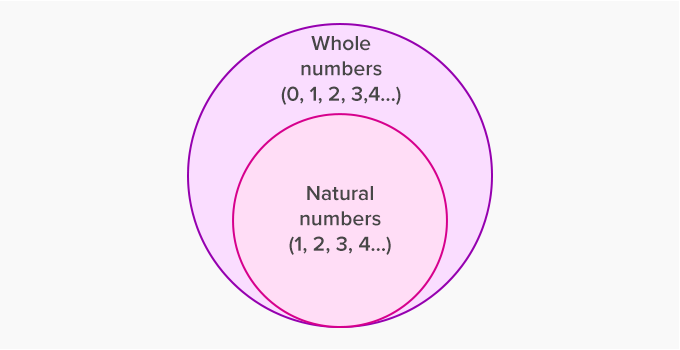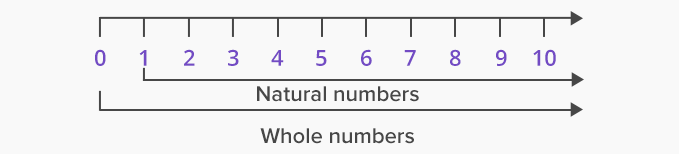# Number - Definition with Examples

The Complete K-5 Math Learning Program Built for Your Child

• 40 Million Kids

Loved by kids and parent worldwide

• 50,000 Schools

Trusted by teachers across schools

• Comprehensive Curriculum

Aligned to Common Core

## What is Numbers?

We count things using numbers.

For example, this is one butterfly and these are 4 butterflies.We start counting things from 1. The numbers 1, 2, 3, 4, ... are called counting numbers or natural numbers.

How do we count something that was there and is no more?

For example, if there were 4 puppies and now there’s none. How do we show none or nothing?

We use Zero (0) to show nothing.

The counting numbers or natural numbers along with zero form whole numbers.We use the digits 0 to 9 to form all the other numbers. Using these 10 digits we can form infinite numbers.For example, 121; 34987; 2987633; 459227904; ...

This number system using 10 digits is called Decimal Number System.

There are many ways in which we can classify numbers.

## Even and Odd Numbers

 Even Numbers Odd Numbers Numbers that end with a 0, 2, 4, 6 or 8 are called even numbers. Example, 14, 202, 500, 8146, 99718 Even numbers are evenly divisible by 2. Example, 244 ÷ 2 = 122 Numbers that end with 1, 3, 5, 7 or 9 are called odd numbers. Example, 11, 213, 6005, 2097, 36739 Odd numbers are not evenly divisible by 2. They always leave a remainder. Example, 245 ÷ 2 gives 122 as quotient and 1 as remainder.

## Prime and Composite Numbers

 Prime Numbers Composite Numbers Numbers that are divisible by only 1 and themselves are called Prime numbers. A prime number has only 2 factors, 1 and itself. Example: 1, 2, 3, 5, 7, 11, 13, 17, 19,23, 29, ... Numbers that are divisible by numbers other than 1 and themselves are called composite numbers. A composite number has more than 2 factors. Example: 4, 6, 8,9, 12, 14, 15, 16, 200, 1122

 Fun Facts Just as natural numbers extend infinitely to the right of 0, numbers extend infinitely to the left of 0 as well. These numbers are called negative numbers. Negative numbers along with whole numbers are called integers. Negative numbers are used to measure temperature, depth, loss, etc.

Won Numerous Awards & Honors